Next: Problems Up: Dirac Equation Previous: Free-Particle Solutions and Wave

# Klein Paradox for Spin-1/2 Particles

Consider the scattering of an electron of energy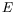and momentum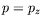by the electrostatic step-potential of the form (figure 5.4)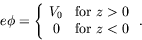(5.279)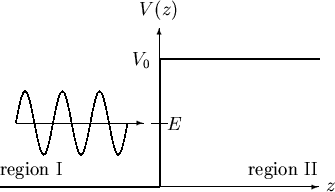For a free electron, we have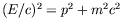, whereas in the presence of the constant potential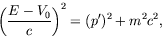(5.280)

where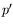is the momentum of the particle inside the potential.

The Dirac equation for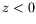is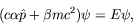(5.281)

while for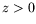we have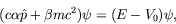(5.282)

where it is understood that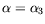and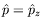.

The incident wave in region I is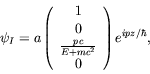(5.283)

with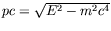. The reflected wave in region I is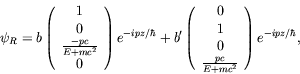(5.284)

The transmitted wave in region II is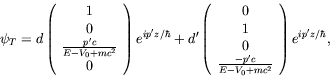(5.285)

with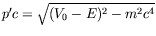.

For now, we consider the case of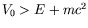(strong field) for which the momentumis real and allows the free plane wave to propagate in region II. Continuity at the boundary requires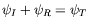and thus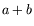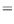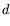(5.286)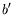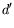(5.287)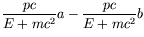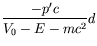(5.288)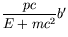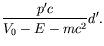(5.289)

The equations for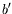and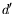can only be satisfied if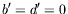. There is thus no spin flip of the electron at the boundary. Also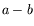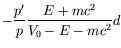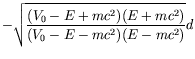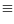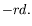(5.290)

We thus have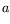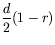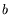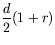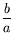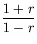(5.291)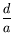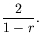(5.292)

The particle current is given by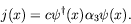(5.293)

We calculate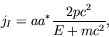(5.294)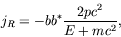(5.295)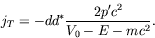(5.296)

Since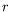is real, the ratio of currents is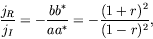(5.297)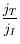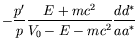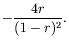(5.298)

Since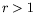, we see that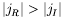. This result corresponds to the fact that the flow of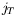is in the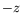-direction, ie. the electrons are leaving region II, but according to our assumptions up to now, there are no electrons in there anyways. A reinterpretation is thus necessary.

To prevent the transition of all electrons to states of negative energy one has to require that all electron states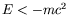are occupied with electrons. The potential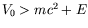raises the electron energy in region II sufficiently for there to be an overlap between the negative continuum forand the positive continuum for, as shown in figure 5.5. In the case ofthe electrons striking the potential barrier from the left are able to knock additional electrons out of the vacuum on the right, leading to positron current flowing from left to right in the potential region. It is possible to understand the sign ofby assuming that the electrons entering region I are coming from the negative continuum.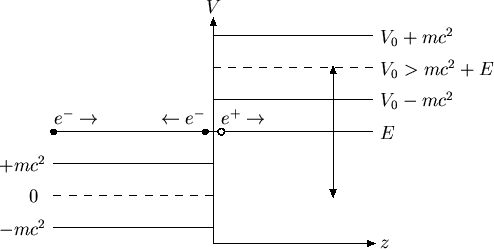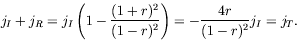(5.299)

Since the holes remaining in region II are interpreted as positrons, the phenomena can be understood as electron-positron pair creation at the potential barrier and is related to the decay of the vacuum in the presence of a strong field.Next: Problems Up: Dirac Equation Previous: Free-Particle Solutions and Wave
Douglas M. Gingrich (gingrich@ ualberta.ca)
2004-03-18